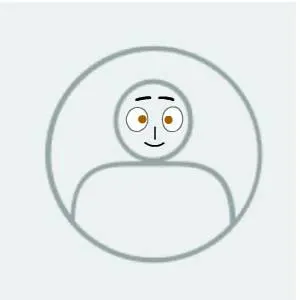Published

# Talking Clock

Using SD Card and 16x2 LCD module.

IntermediateFull instructions provided9,696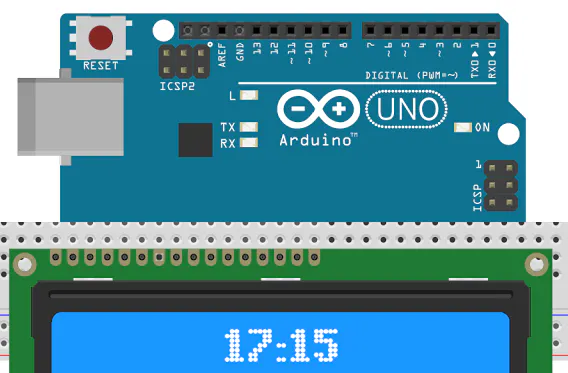## Things used in this project

### Hardware components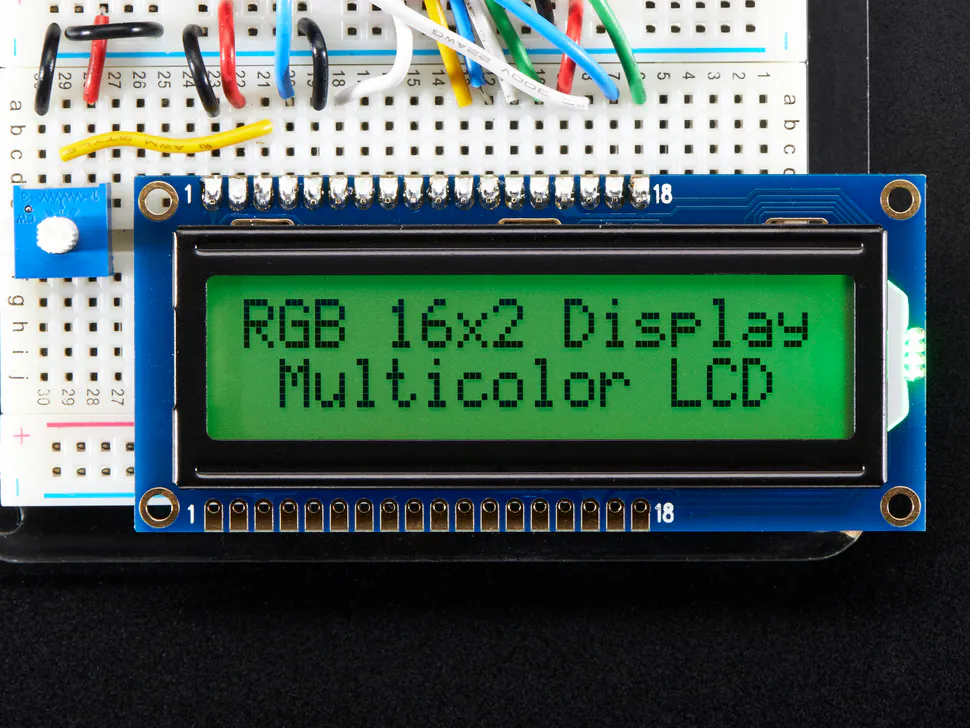Adafruit RGB Backlight LCD - 16x2
×1Arduino UNO
×1Resistor 1k ohm
×3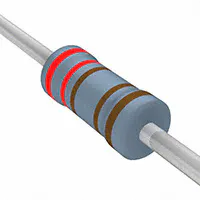Resistor 2.21k ohm
×3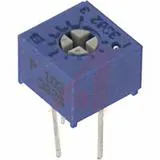Single Turn Potentiometer- 10k ohms
×1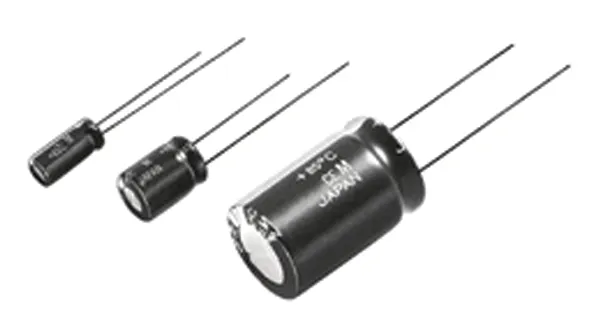Capacitor 22 µF
×2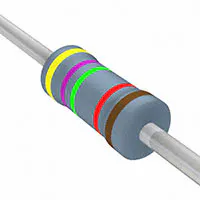Resistor 47.5k ohm
×1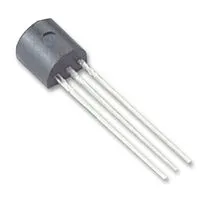General Purpose Transistor NPN
×1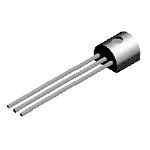General Purpose Transistor PNP
×1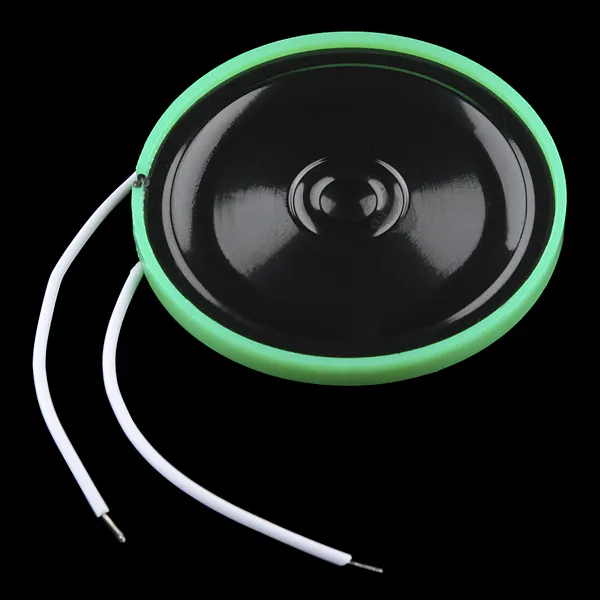Speaker: 0.25W, 8 ohms
×1SparkFun Pushbutton switch 12mm
×3
 SD Socket
×1

## Schematics

### arduino clock2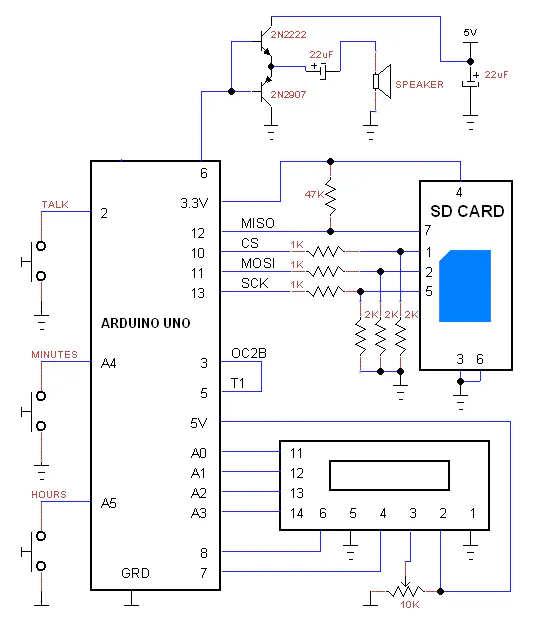## Code

### Talking Clock

C/C++
```/*
* sd_bell.ino
*
* Created: 5/06/2017 23:34:47
*  Author: moty22.co.uk
*/

#include <LiquidCrystal.h>

// initialize the library with the numbers of the interface pins
LiquidCrystal lcd(7, 8, A0, A1, A2, A3);

const int CS = 10;          // SD CS connected to digital pin PD4
const int mosi = 11;
const int clk = 13;
const int ampON = 9;          // led connected to digital pin PB0
const int audio = 6;     //output D6
const int oc2b = 3;     //1000Hz
const int t1 = 5;     //1000Hz
const int talk = 2;     //talk pushbutton
const int minutes = A4;     //minutes advance pushbutton
const int hours = A5;     //hours pushbutton
int talkPB=1;         // talk pushbutton status
int minutesPB=1;         // minutes pushbutton status
int hoursPB=1;         // hours pushbutton status

unsigned long loc,BootSector, RootDir, SectorsPerFat, RootDirCluster, DataSector, FileCluster, FileSize;  //
unsigned int BytesPerSector, ReservedSectors, card;
unsigned char fn=1, sdhc=0, SectorsPerCluster, Fats;
unsigned char minute=0, hour=0;

void setup() {
// put your setup code here, to run once:
pinMode(CS, OUTPUT);
pinMode(mosi, OUTPUT);
pinMode(clk, OUTPUT);
pinMode(ampON, OUTPUT);
pinMode(talk, INPUT_PULLUP);
pinMode(minutes, INPUT_PULLUP);
pinMode(hours, INPUT_PULLUP);

lcd.begin(16, 2);   // set up the LCD's number of columns and rows:

//spi init
SPCR = _BV(SPE) | _BV(MSTR) | _BV(SPR0) | _BV(SPR1);  // Enable spi, Master, set clock rate fck/64
SPSR = _BV(SPI2X);    //set clock rate fck/64 = 250KHz

//PWM Timer0
OCR0A = 0;
TCCR0A = _BV(COM0A1) | _BV(WGM01) | _BV(WGM00);  //output in phase, fast PWM mode
TCCR0B = _BV(CS00); // 16MHz/256 = 62.5KHz
pinMode(audio, OUTPUT);

//1000 Hz - timer2
OCR2A =249;
OCR2B = 125;
TCCR2A = _BV(COM2B1) | _BV(COM2B0) | _BV(WGM21) | _BV(WGM20);  //output B in phase, fast PWM mode
TCCR2B = _BV(WGM22) | _BV(CS22); // set prescaler to 64 and start the timer
pinMode(oc2b, OUTPUT);

//60 sec - timer1
// OCR1A = 0xEA5F;
TCCR1A = _BV(WGM10) | _BV(WGM11) | _BV(COM1A0); //
TCCR1B = _BV(WGM12) | _BV(WGM13) | _BV(CS11) | _BV(CS12);  // input T1, PWM mode

digitalWrite(CS, HIGH);
//digitalWrite(ampON, LOW);

InitSD();
if(sdhc){card = 1;}else{card=512;}  //SD or SDHC
fat();
if(BytesPerSector!=512){error();}

}

void loop()
{

if(talkPB==LOW)
{
if(hour < 21) {fn=hour; play();}
else if(hour == 20) {fn=20; play();}
else if(hour > 20) {fn=20; play(); fn=hour-20; play();}

if(minute < 21) {fn=minute; play();}
else if(minute == 20) {fn=20; play();}
else if(minute > 20 && minute < 30) {fn=20; play(); fn=minute-20; play();}
else if(minute == 30) {fn=21; play();}
else if(minute > 30 && minute < 40) {fn=21; play(); fn=minute-30; play();}
else if(minute == 40) {fn=22; play();}
else if(minute > 40 && minute < 50) {fn=22; play(); fn=minute-40; play();}
else if(minute == 50) {fn=23; play();}
else if(minute > 50 && minute < 60) {fn=23; play(); fn=minute-50; play();}
OCR0A=0;    //audio off

}

if(minutesPB==LOW)
{
minute++;
if(minute>59) {minute=0;}
display();
wait();
}

if(hoursPB==LOW)
{
hour++;
if(hour>23) {hour=0;}
display();
wait();
}

if(TIFR1 & _BV(OCF1A))   //1 minute elapsed
{
OCR1A = 0xEA5F;  //59999 counts = 1 minute
TIFR1 = _BV(OCF1A);  //TMR1 interrupt reset

minute++;
if(minute>59) {minute=0; hour++;}
if(hour>23) hour=0;
display();

}
}

//find the file and play it
void play(void)
{
if(!sdhc){fn += 1;}  //if not SDHC first file = 1
if(fn > 15)
{
fn -=16; file(fn*32+20,1);    //32 bytes per file descriptor at offset of 20
}
else
{
file(fn*32+20,0);    //32 bytes per file descriptor at offset of 20
}
loc=(1 + (DataSector) + (unsigned long)(FileCluster-2) * SectorsPerCluster) * card ;
}

//LCD update
void display(void)
{
lcd.setCursor(5, 0); // top left
if(hour<10)lcd.print(" ");
lcd.print(hour);
lcd.print(":");
if(minute<10)lcd.print("0");
lcd.print(minute);
}

//SD error display
void error(void)
{
lcd.setCursor(0, 1); // bottom left
lcd.print("SD ERROR");
lcd.setCursor(0, 0); // top left
}

//1 sec delay
void wait(void)
{
unsigned long i; //,j
for(i=0;i<100000;i++)
{
digitalWrite(ampON, LOW);
}
}

{
unsigned int i,r;
digitalWrite(CS, LOW);
if(r != 0) error();     //if command failed error();

while(FileSize--)
{
while(spi(0xFF) != 0xFE); // wait for first byte
for(i=0;i<512;i++){
// 3 rounds of timer 0 = total of time to get 22 khz
while (!(TIFR0 & _BV(OCF0A))){}
TIFR0 = _BV(OCF0A);  //TMR0 interrupt reset
while (!(TIFR0 & _BV(OCF0A))){}
TIFR0 = _BV(OCF0A);  //TMR0 interrupt reset
while (!(TIFR0 & _BV(OCF0A))){}

OCR0A=spi(0xFF);    //write byte to PWM
TIFR0 = _BV(OCF0A);  //TMR0 interrupt reset
}
spi(0xFF);
}

Command(12,0x00,0xFF);  //stop transmit
spi(0xFF);
spi(0xFF);
digitalWrite(CS, HIGH);
spi(0xFF);
}

void file(unsigned int offset, unsigned char sect)  //find files
{
unsigned int r,i;
unsigned char fc, fs; //

digitalWrite(CS, LOW);
r = Command(17,(RootDir+sect)*card,0xFF);   //read boot-sector for info from file entry
if(r != 0) error();     //if command failed

while(spi(0xFF) != 0xFe); // wait for first byte
for(i=0;i<512;i++){
if(i==offset){fc=spi(0xFF);}
else if(i==offset+1){fc=spi(0xFF);}
else if(i==offset+6){fc=spi(0xFF);}
else if(i==offset+7){fc=spi(0xFF);}

else if(i==offset+8){fs=spi(0xFF);}
else if(i==offset+9){fs=spi(0xFF);}
else if(i==offset+10){fs=spi(0xFF);}
else if(i==offset+11){fs=spi(0xFF);}
else{spi(0xFF);}

}
spi(0xFF);
digitalWrite(CS, HIGH);
spi(0xFF);
FileCluster = fc | ( (unsigned long)fc << 8 ) | ( (unsigned long)fc << 16 ) | ( (unsigned long)fc << 24 );
FileSize = fs | ( (unsigned long)fs << 8 ) | ( (unsigned long)fs << 16 ) | ( (unsigned long)fs << 24 );
FileSize = FileSize/512-1;    //file size in sectors
}

void fat (void)
{
unsigned int r,i;
unsigned char pfs,bps1,bps2,rs1,rs2,spf,rdc; //pfs=partition first sector ,de1,de2,spf1,d

digitalWrite(CS, LOW);
if(r != 0) error();     //if command failed

while(spi(0xFF) != 0xFe); // wait for first byte
for(i=0;i<512;i++){
if(i==454){pfs=spi(0xFF);} //pfs=partition first sector
else if(i==455){pfs=spi(0xFF);}
else if(i==456){pfs=spi(0xFF);}
else if(i==457){pfs=spi(0xFF);}
else{spi(0xFF);}

}
spi(0xFF);
digitalWrite(CS, HIGH);
spi(0xFF);
//convert 4 bytes to long int
BootSector = pfs | ( (unsigned long)pfs << 8 ) | ( (unsigned long)pfs << 16 ) | ( (unsigned long)pfs << 24 );

digitalWrite(CS, LOW);
if(r != 0) error();     //if command failed

while(spi(0xFF) != 0xFe); // wait for first byte
for(i=0;i<512;i++){

if(i==11){bps1=spi(0xFF);} //bytes per sector
else if(i==12){bps2=spi(0xFF);}
else if(i==13){SectorsPerCluster=spi(0xFF);}
else if(i==14){rs1=spi(0xFF);}
else if(i==15){rs2=spi(0xFF);}
else if(i==16){Fats=spi(0xFF);} //number of FATs
else if(i==36){spf=spi(0xFF);}
else if(i==37){spf=spi(0xFF);}
else if(i==38){spf=spi(0xFF);}
else if(i==39){spf=spi(0xFF);}
else if(i==44){rdc=spi(0xFF);}
else if(i==45){rdc=spi(0xFF);}
else if(i==46){rdc=spi(0xFF);}
else if(i==47){rdc=spi(0xFF);}
else{spi(0xFF);}

}
spi(0xFF);
digitalWrite(CS, HIGH);
spi(0xFF);

BytesPerSector = bps1 | ( (unsigned int)bps2 << 8 );
ReservedSectors = rs1 | ( (unsigned int)rs2 << 8 ); //from partition start to first FAT
RootDirCluster = rdc | ( (unsigned long)rdc << 8 ) | ( (unsigned long)rdc << 16 ) | ( (unsigned long)rdc << 24 );
SectorsPerFat = spf | ( (unsigned long)spf << 8 ) | ( (unsigned long)spf << 16 ) | ( (unsigned long)spf << 24 );
DataSector = BootSector + (unsigned long)Fats * (unsigned long)SectorsPerFat + (unsigned long)ReservedSectors;  // + 1
RootDir = (RootDirCluster -2) * (unsigned long)SectorsPerCluster + DataSector;
}

unsigned char spi(unsigned char data)   // send character over spi
{
SPDR = data;  // Start transmission
while(!(SPSR & _BV(SPIF)));   // Wait for transmission to complete

}
//assemble a 32 bits command
char Command(unsigned char frame1, unsigned long adrs, unsigned char frame2 )
{
unsigned char i, res;
spi(0xFF);
spi((frame1 | 0x40) & 0x7F);  //first 2 bits are 01
spi(frame2 | 1);        //CRC and last bit 1

for(i=0;i<10;i++) // wait for received character
{
res = spi(0xFF);
if(res != 0xFF)break;
}
return res;
}

void InitSD(void)
{
unsigned char i,r;

digitalWrite(CS, HIGH);
for(i=0; i < 10; i++)spi(0xFF);   // min 74 clocks
digitalWrite(CS, LOW);      // Enabled for spi mode

i=100;  //try idle state for up to 100 times
while(Command(0x00,0,0x95) !=1 && i!=0)
{
digitalWrite(CS, HIGH);
spi(0xFF);
digitalWrite(CS, LOW);
i--;
}
if(i==0)  error(); //idle failed

if (Command(8,0x01AA,0x87)==1){         //check card is 3.3V
r=spi(0xFF); r=spi(0xFF); r=spi(0xFF); r=spi(0xFF);   //rest of R7
if ( r == 0x01 && r == 0xAA ){    //Vdd OK (3.3V)

//Command(59,0,0xFF);   //CRC off
Command(55,0,0xFF);
while(Command(41,0x40000000,0xFF)){Command(55,0,0xFF);}   //ACMD41 with HCS bit
}
}else{error();}

if (Command(58,0,0xFF)==0){   //read CCS in the OCR - SD or SDHC
r=spi(0xFF); r=spi(0xFF); r=spi(0xFF); r=spi(0xFF);   //rest of R3
sdhc=r & 0x40;
//if(r & 0x40)sdLED=1;
}
SPCR &= ~(_BV(SPR1));  // set clock rate fck/8 = 2MHz
digitalWrite(CS, HIGH);
}
```

## Credits

### moty

12 projects • 80 followers